﻿ Contact-Boundary Value Problem in the Non-Classical Treatment for One Pseudo-Parabolic Equation

### Contact-Boundary Value Problem in the Non-Classical Treatment for One Pseudo-Parabolic Equation

Ilgar G. MamedovOPEN ACCESSPEER-REVIEWED

## Contact-Boundary Value Problem in the Non-Classical Treatment for One Pseudo-Parabolic Equation

Ilgar G. MamedovInstitute of Cybernetics Azerbaijan National Academy of Sciences, Baku, Azerbaijan

### Abstract

In this paper substantiated for a differential equation of pseudo-parabolic type with discontinuous coefficients a contact-boundary value problem with non-classical boundary conditions is considered, which requires no matching conditions. Equivalence of these conditions boundary condition is substantiated classical, in the case if the solution of the problem in the izotropic S.L. Sobolev's space is found. The considered equation as a pseudo-parabolic equation generalizes not only classic equations of mathematical physics (heat-conductivity equations, string vibration equation) and also many models differential equations (Aller's equation, Manjeron equation, telegraph equation, moisture transfer generalized equation, Boussinesq - Love equation and etc.). It is grounded that the contact-boundary conditions in the classic and non-classic treatment are equivalent to each other, and such boundary conditions are demonstrated in geometric form. Even from geometric interpretation can see that the grounded non-classic treatment doesn't require any additional conditions of agreement type. Thus, namely in this paper, the non-classic problem with contact-boundary conditions is grounded for a pseudo-parabolic equation. For simplicity, this was demonstrated for one model case in one of S.L. Sobolev izotropic space Wp(4,4)(G).

### At a glance: Figures

1
Prev Next

• Mamedov, Ilgar G.. "Contact-Boundary Value Problem in the Non-Classical Treatment for One Pseudo-Parabolic Equation." Applied Mathematics and Physics 2.2 (2014): 49-52.
• Mamedov, I. G. (2014). Contact-Boundary Value Problem in the Non-Classical Treatment for One Pseudo-Parabolic Equation. Applied Mathematics and Physics, 2(2), 49-52.
• Mamedov, Ilgar G.. "Contact-Boundary Value Problem in the Non-Classical Treatment for One Pseudo-Parabolic Equation." Applied Mathematics and Physics 2, no. 2 (2014): 49-52.

 Import into BibTeX Import into EndNote Import into RefMan Import into RefWorks

### 1. Introduction

Pseudo-parabolic equations are attracted for sufficiently adequate description of a great deal of real processes occurring in the nature, engineering and etc. In particular, many processes arising in the theory of fluid filtration in cracked media are described by discontinuous coefficient pseudo-parabolic equations.

Urgency of investigations conducted in this field is explained by appearance of local and non-local problems for discontinuous coefficients equations connected with different applied problems. Such type problems arise for example, while studying the problems of moisture, transfer in soils, heat transfer in heterogeneous media, diffusion of thermal neutrons in inhibitors, simulation of different biological processes, phenomena and etc. In the present paper, here consider contact-boundary value problem for one pseudo-parabolic equation with discontinuous coefficients. The coefficients in this pseudo-parabolic equation are not necessarily differentiable; therefore, there does not exist a formally adjoint differential equation making a certain sense. For this reason, this question cannot be investigated by the well-known methods using classical integration by parts and Riemann functions or classical-type fundamental solutions. The theme of the present paper, devoted to the investigation contact-boundary value problem for differential equations of pseudo-parabolic type, according to the above-stated is very actual for the solution of theoretical and practical problems. From this point of view, the paper is devoted to the actual problems of applied mathematics and physics.

### 2. Problem Statement

Consider equation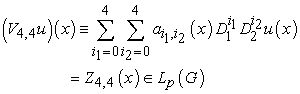(1)

where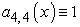.

Here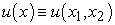is a desired function determined on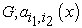are the given measurable functions on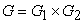, where.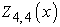is a given measurable function on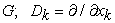is a generalized differentiation operator in S.L. Sobolev sense,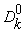is an identity transformation operator.

Equation (1) is a hyperbolic equation that has two real characteristics, the first and second one of which is four-fold. Therefore, we can consider equation (1) in some sense as a pseudo-parabolic equation . This equation is a Boussinesq - Love generalized equation from the vibrations theory  and Aller's equation under mathematical modeling [, p.261] of the moisture absorption process in biology.

In the paper, we consider equation (1) in the general case when the coefficients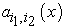are non smooth functions satisfying the following conditions: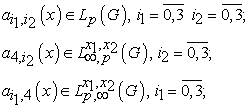Therewith, the important principal moment is that the equation under consideration has discontinuous coefficients that satisfy only some p-integrability and boundedness conditions, i.e. the considered pseudo-parabolic differential operator has no traditional conjugation operator.

Under these conditions, we'll look for the solution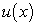of equation (1) in S.L. Sobolev isotropic space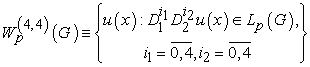where.

For equation (1) we can give the contact-boundary conditions of the classic form as follows: (see Figure 1 ):

Figure 1. Geometric interpretation of classic contact-boundary conditions(2)

whereare the given measurable functions on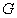. It is obvious that in the case of conditions (2), in addition to the conditions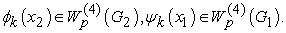the given functions should satisfy also the following agreement conditions: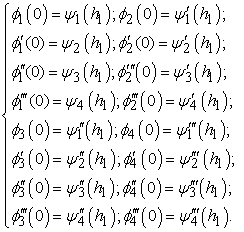(3)

Consider the following non-classical boundary conditions:(4)

### 3. Methodology

There with, the important principal moment is that the considered equation possesses discontinuous coefficients satisfying only some P -integrability and boundedness conditions i.e. the considered pseudo-parabolic operator has no traditional conjugated operator. In other words, the Riemann function for this equation can't be investigated by the classical method of characteristics. In the papers [4, 5] the Riemann function is determined as the solution of an integral equation. This is more natural than the classical way for deriving the Riemann function. The matter is that in the classic variant, for determining the Riemann function, the rigid smooth conditions on the coefficients of the equation are required.

The Riemann’s method does not work for differential equations with discontinuous coefficients.

In the present paper, a method that essentially uses modern methods of the theory of functions and functional analysis is worked out for investigations of such problems. In the main, this method it requested in conformity to pseudo-parabolic equations. Notice that, in this paper the considered equation is a generation of many model equations of some processes (for example, Aller's equation, Manjeron equation, telegraph equation, heat-conductivity equations, moisture transfer generalized equation, string vibrations equations and etc).

If the function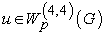is a solution of the classical form contact boundary value problem (1), (2), then it is also a solution of problem (1), (4) for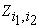, determined by the following equalities: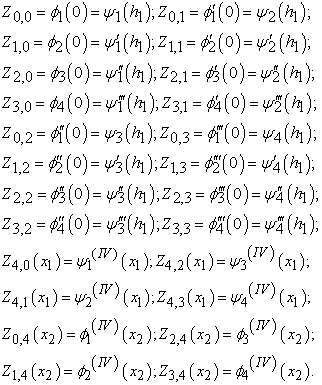It is easily proved that the inverse is also true. In other words, if the functionis a solution of problem (1), (4), it is also a solution of problem (1), (2) for the following functions:(5)(6)(7)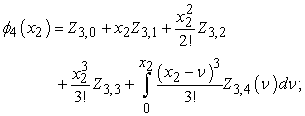(8)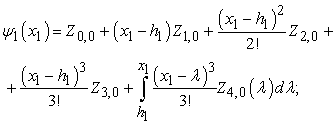(9)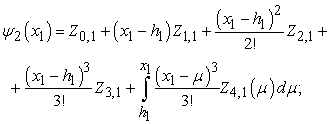(10)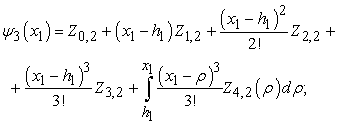(11)(12)
Figure 2. Geometric interpretation of contact-boundary value conditions in non-classical treatment

Note that the functions (5)-(12) possess an important property, more exactly, the agreement conditions for all, possessing the above-mentioned properties are fulfilled for them. Therefore, equalities (5)-(12) may be considered as a general form of all the functionssatisfying agreement conditions (3).

### 4. Result

So, the classic form contact-boundary value problem (1), (2) and in non-classic treatment (1), (4) (see. Figure 2) are equivalent in the general case. However, the contact-boundary value problem in non-classic treatment (1), (4) is more natural by the statement than problem (1), (2). This is connected with the fact that in the statement of problem (1), (4), the right sides of boundary conditions don’t require additional conditions of agreement type. Note that some boundary value problems in non-classic treatments were considered in the author’s papers [6, 7, 8, 9, 10].

### 5. Discussion and Conclusions

In the paper a non-classical type contact-boundary value problem is substantiated for a pseudo-parabolic equation with non-smooth coefficients and with dominating derivative. Classic contact-boundary conditions are reduced to non-classic contact-boundary conditions by means of integral representations. Such statement of the contact-boundary problem has several advantages:

1. No additional agreement conditions are required in this statement;

2. One can consider this statement as a contact-boundary value problem formulated in terms of traces in the S.L. Sobolev izotropic space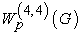;

3. In this statement the considered equation is a generalization of many model equations of some processes (e.g. Aller's equation, Manjeron equation, telegraph equation, heat-conductivity equations, moisture transfer generalized equation, Boussinesq-Love equation, string vibrations equations and etc.).

### References

  D. Colton, Pseudo-parabolic equations in one space variable, Different. Equations, vol. 12, No. 3, (1972), 559-565.In article CrossRef  A.P. Soldatov, M.Kh. Shkhanukov, Boundary value problems with A.A. Samarsky general nonlocal condition for higher order pseudo-parabolic equations, Dokl. AN SSSR, vol. 297, No. 3, (1987), 547-552 (in Russian).In article  A.M. Nakhushev, Equations of mathematical biology, M.: Visshaya Shkola, 301p, 1995 (in Russian).In article  S.S. Akhiev, Fundamental solution to some local and non - local boundary value problems and their representations, DAN USSR, vol.271, No 2, (1983), 265-269 (in Russian).In article  V.I. Zhegalov, E.A. Utkina, on a third order pseudo-parabolic equation, Izv. Vuzov, Matem. No 10, (1999), 73-76 (in Russian).In article  I.G. Mamedov, A fundamental solution to the Cauchy problem for a fourth-order pseudo-parabolic equation, Springer, Computational Mathematics and Mathematical Physics, vol. 49, Issue 1, (2009), 93-104.In article CrossRef  I.G. Mamedov, Goursat non - classic three dimensional problems for a hyperbolic equation with discontinuous coefficients, Vestnik Samarskogo Gosudarstvennogo Tekhnicheskogo Universiteta, Ser. Fiz.-Mat. Nauki, No. 1 (20), (2010), 209-213 (in Russian).In article  I.G. Mamedov, Fundamental solution of initial boundary value problem for a fourth order pseudo-parabolic equation with non-smooth coefficients, Vladikavkazskii Matematicheskii Zhurnal, vol. 12, No 1, (2010), 17-32 (in Russian).In article  I.G. Mamedov, A non-classical formula for integration by parts related to Goursat problem for a pseudo-parabolic equation, Vladikavkazskii Matematicheskii Zhurnal, vol. 13, No 4, (2011), 40-51 (in Russian).In article  I.G. Mamedov, Contact-boundary value problem for a hyperbolic equation with multiple characteristics, Functional analysis and its applications, Proceedings of the International Conference devoted to the centenary of academician Z. I. Khalilov, Baku, (2011), 230-232 (in Russian).In article